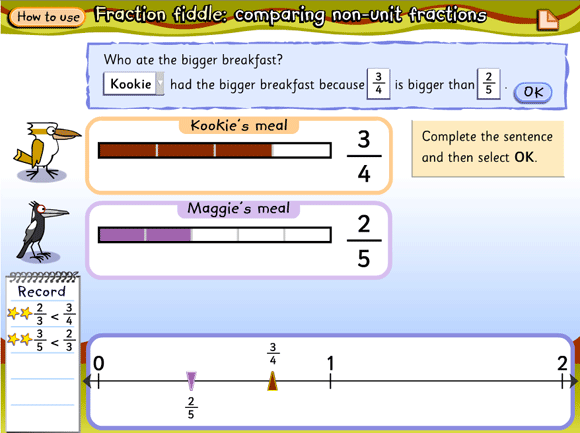Home > Fractions > Misunderstandings > Using rules blindly > Comparing non-unit fractions

# Comparing non-unit fractions

Display the learning object L2803 Fraction fiddle: comparing non-unit fractions to the class on an interactive whiteboard (or projector and screen).Screen grab from L2803 Fraction fiddle: comparing non-unit fractions.
Source: © Education Services Australia Ltd, 2011

• Work through a task together, pausing for students to make predictions.
•  Which fraction do you think is smaller/larger?
•  Why do you think that?
• Encourage students to notice the relationships between the numerator/denominator and the construction of the fraction bar.
• What happens to the bar as the denominator gets larger?
• What happens to the bar as the numerator gets larger?
• Encourage students to notice the position of the fractions on the number line.
• Look where $$\frac{3}{4}$$ is located. Where would $$\frac{1}{4}$$ be? What about $$\frac{1}{2}$$?
• Is $$\frac{2}{5}$$ going to be closer to 1 than $$\frac{3}{4}$$ or further away? Why do you think so?

Students can work in pairs at computers to complete a set of tasks. The printed record of their findings can be the basis of further discussions about strategies for solving similar tasks without the learning object.

• What can you do to help you work out which fraction is larger or smaller?
• Why can’t you decide just by looking at the denominators?